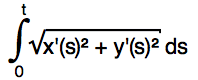Posted by: pixelero | July 5, 2011

## Pixel Bender: SplineAlignedImage – the Implicit Approach

In principle this is the same effect I already did with html5/canvas. Now it’s time for Pixel Bender to try some serious image bending.Click on the image above to go to the demo.

Ok, drawing a spline, or more generally rendering graphics along a spline, is relatively simple if you can explicitly calculate points of it and draw it part by part using lines, gradients or piecies of an image.
But it all becomes much more challening when in an environment, like Pixel Bender, where you can only go through the image pixel by pixel – and take the implicit approach.
If we where dealing with a line or a circle, calculating the distance to it would be so easy, but points on a Bezier are defined by a parametric cubic polynomial, and there’s no way to solve the inverse process algebrally. So what to do? There has to be some way to map the screen coordinates to a Bezier system:Ok, looking at a picture always helps. Let’s say our current point is the black dot on picture and the Bezier passes on the top of the grid as the blue line.  First we find the nearest point on the curve, below ‘x’ where blue and red line meet. Distance to that, the red line, would be the new coordinate y. Then we calculate the distance along the path to that nearest point (blue line), length corresponds to coordinate x. Both of these calculation are ‘impossible’ to write as single evaluations, so we need some approximation.From the curve we know the start and end points and the directions at both, so let’s start with this piece of information. We count, using dot product of two vectors, the cosine of angles between directions and the distance vectors to current point. If this value has different signs on the ends, current point lies somewhere in between, and we’re looking for the point where the value goes to zero.

Using values the start and end we approximate a point in between, repeating this a couple of times converges to a point where cos γ ≈ 0.0. For most cases this is a reliable result, although we totally neglected the fact that Beziers can even form closed loops, those cases would require splitting the curve to several sequences by points where x”=0 or y”=0. I mean the code should check out three candidates for the closest point.

My pixel bender-kernel repeates the iteration three times, which I think is adequate enough for the purpose of a computer graphics demonstration. In fact the method slightly differs from the principle I described, that’s only because also wanted to add the effect for rotating the image around spline’s main axis.

The mystery to solve here is a system of two nonlinear equations. You could also try solving that with like for instance Euler iteration using Jacobian determinate. The problem with that method is that it easily tends to converge to unwanted solutions, to points somewhere far from the actual spline sequence. Remember that Bezier is a continuous curve, where we only see the sequence from t=0 to t=1.

Ok, back to task. We now have the distance ‘y’. We need also the ‘x’, so all we need to do is just to calculate the distance from start point:But now wait a minute, that’s an Elliptic integral or something else as awful — unfortunately there’s no build-in function for it in Pixel Bender.  So we’ll use Simpson’s rule to get some idea of the length. In the simpliest case of that we calculate the sum of (value at start +3x (values at point of one third+value at point of two third) + value at end)/8. And to my surprise looking at the result it is enough!
I looked at the result with a more precise approach: sampled at 7 points with weights 1,3,3,2,3,3,1 – visually there was hardly any difference. In the demo same method is used when fitting an image’s width to spline’s length. It is said that Simpson perfectly approximates a cubic polynomial. In the formula x(t) and y(t) are cubic polynomials, which makes x'(t)² + y'(t)² of degree four, and square root of that could be something close to quadratic – at least that’s how I explained it to myself.As a conclusion. This is probably the stupidiest way of drawing by a spline: There are still errors in more ‘knotty’ cases. Furthermore the shader has to go through the entire bitmap to render even a smallest curve.

At least inside Flash Player much better results are obvious obtained by using methods of graphics like in my earlier post in case of 2D. Anyway I wanted to post it here, even if nothing else as an example of solving some awful mathematics.## Responses

1.Fantastic work!! And very solid results… Thanks for sharing the sources, this is a very valuable resource.

2.Hi,
I really like your blog posts but i wish you had an rss feed so that i could follow you!

3.crazy!

4.Hi, I have an adobe flash builder project that take ID pictures and I need to make the background to became completly white. I was necessary to adjuste the white balance of the picture. Do you think you can do that?

5. […] Base from: Pixelero […]